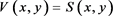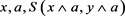﻿ 有界格上的Null–范数构造方法的讨论

# 有界格上的Null–范数构造方法的讨论Discussion on the Construction of Null-Norms on Bounded Lattices

Abstract: Based on the concept of null-norm on bounded lattices, this paper gives a theorem that indicates there are only three construction methods of null-norm on bounded lattices, and gives a proposition that points out a relationship of the three null-norms with fixed zero element a.

1. 引言

2. 预备知识

$\left(L,\le ,0,1\right)$ 是一个有界格， $a\in L$，那么与a不可比的元素的集合我们记作 ${I}_{a}=\left\{x\in L|x||a\right\}$

1) $T\left(x,y\right)=T\left(y,x\right)$ (交换性)；

2) $T\left(T\left(x,y\right),z\right)=T\left(x,T\left(y,z\right)\right)$ (结合性)；

3) 当 $y\le z$ 时，有 $T\left(x,y\right)\le T\left(x,z\right)$ (单调递增性)；

4) $T\left(x,1\right)=1$

1) $S\left(x,y\right)=S\left(y,x\right)$ (交换性)；

2) $S\left(S\left(x,y\right),z\right)=S\left(x,S\left(y,z\right)\right)$ (结合性)；

3) 当 $y\le z$ 时，有 $S\left(x,y\right)\le S\left(x,z\right)$ (单调递增性)；

4) $S\left(x,0\right)=x$

1) $V\left(x,y\right)=V\left(y,x\right)$ (交换性)；

2) $V\left(V\left(x,y\right),z\right)=V\left(x,V\left(y,z\right)\right)$ (结合性)；

3) 当 $y\le z$ 时，有 $V\left(x,y\right)\le V\left(x,z\right)$ (单调递增性)；

4) 存在 $a\in \left[0,1\right]$，对任意的 $x\in \left[0,a\right]$，使得 $V\left(x,0\right)=x$ ；对任意的 $x\in \left[a,1\right]$，使得 $V\left(x,1\right)=x$

${T}_{W}\left(x,y\right)=\left\{\begin{array}{l}y\text{\hspace{0.17em}}\text{\hspace{0.17em}}\text{\hspace{0.17em}}\text{\hspace{0.17em}}\text{\hspace{0.17em}}\text{\hspace{0.17em}}x=1\\ x\text{\hspace{0.17em}}\text{\hspace{0.17em}}\text{\hspace{0.17em}}\text{\hspace{0.17em}}\text{\hspace{0.17em}}\text{\hspace{0.17em}}y=1\\ 0\text{\hspace{0.17em}}\text{\hspace{0.17em}}\text{\hspace{0.17em}}\text{otherwise}\end{array}$

${T}_{\wedge }\left(x,y\right)=x\wedge y$

${S}_{\vee }\left(x,y\right)=x\vee y$

${S}_{W}\left(x,y\right)=\left\{\begin{array}{l}y\text{\hspace{0.17em}}\text{\hspace{0.17em}}\text{\hspace{0.17em}}\text{\hspace{0.17em}}\text{\hspace{0.17em}}\text{\hspace{0.17em}}x=0\\ x\text{\hspace{0.17em}}\text{\hspace{0.17em}}\text{\hspace{0.17em}}\text{\hspace{0.17em}}\text{\hspace{0.17em}}\text{\hspace{0.17em}}y=0\\ 1\text{\hspace{0.17em}}\text{\hspace{0.17em}}\text{\hspace{0.17em}}\text{otherwise}\end{array}$

2015年，F. Karacal, M.A. Ince, R. Mesiar在文献  中提出了三种Null–范数的构造方法，具体如下：

${V}_{a}^{\left(S\right)}\left(x,y\right)=\left\{\begin{array}{l}S\left(x,y\right)\text{\hspace{0.17em}}\text{\hspace{0.17em}}\text{\hspace{0.17em}}\text{\hspace{0.17em}}\text{\hspace{0.17em}}\text{\hspace{0.17em}}\text{\hspace{0.17em}}\text{\hspace{0.17em}}\text{\hspace{0.17em}}\text{\hspace{0.17em}}\text{\hspace{0.17em}}\text{\hspace{0.17em}}\text{\hspace{0.17em}}\text{\hspace{0.17em}}\text{\hspace{0.17em}}\text{\hspace{0.17em}}\text{\hspace{0.17em}}\text{\hspace{0.17em}}\text{\hspace{0.17em}}\text{\hspace{0.17em}}\text{\hspace{0.17em}}\text{\hspace{0.17em}}\text{\hspace{0.17em}}\text{\hspace{0.17em}}\text{\hspace{0.17em}}\text{\hspace{0.17em}}\text{\hspace{0.17em}}\text{\hspace{0.17em}}\left(x,y\right)\in {\left[0,a\right]}^{2}\\ a\text{\hspace{0.17em}}\text{\hspace{0.17em}}\text{\hspace{0.17em}}\text{\hspace{0.17em}}\text{\hspace{0.17em}}\text{\hspace{0.17em}}\text{\hspace{0.17em}}\text{\hspace{0.17em}}\text{\hspace{0.17em}}\text{\hspace{0.17em}}\text{\hspace{0.17em}}\text{\hspace{0.17em}}\text{\hspace{0.17em}}\text{\hspace{0.17em}}\text{\hspace{0.17em}}\text{\hspace{0.17em}}\text{\hspace{0.17em}}\text{\hspace{0.17em}}\text{\hspace{0.17em}}\text{\hspace{0.17em}}\text{\hspace{0.17em}}\text{\hspace{0.17em}}\text{\hspace{0.17em}}\text{\hspace{0.17em}}\left(x,y\right)\in {\left[a,1\right)}^{2}\cup \left[a,1\right]×{I}_{a}\cup {I}_{a}×\left[a,1\right]\cup {D}_{\alpha }\\ S\left(x\wedge a,y\wedge a\right)\text{\hspace{0.17em}}\text{\hspace{0.17em}}\text{\hspace{0.17em}}\text{\hspace{0.17em}}\text{\hspace{0.17em}}\text{\hspace{0.17em}}\text{ }\left(x,y\right)\in \left[0,a\right]×{I}_{a}\cup {I}_{a}×\left[0,a\right]\cup {I}_{a}×{I}_{a}\\ x\wedge y\text{\hspace{0.17em}}\text{\hspace{0.17em}}\text{\hspace{0.17em}}\text{\hspace{0.17em}}\text{\hspace{0.17em}}\text{\hspace{0.17em}}\text{\hspace{0.17em}}\text{\hspace{0.17em}}\text{\hspace{0.17em}}\text{\hspace{0.17em}}\text{\hspace{0.17em}}\text{\hspace{0.17em}}\text{\hspace{0.17em}}\text{\hspace{0.17em}}\text{\hspace{0.17em}}\text{\hspace{0.17em}}\text{\hspace{0.17em}}\text{\hspace{0.17em}}\text{\hspace{0.17em}}\text{\hspace{0.17em}}\text{\hspace{0.17em}}\text{\hspace{0.17em}}\text{\hspace{0.17em}}\text{\hspace{0.17em}}\text{\hspace{0.17em}}\text{\hspace{0.17em}}\text{\hspace{0.17em}}\text{\hspace{0.17em}}\text{\hspace{0.17em}}\text{\hspace{0.17em}}\text{\hspace{0.17em}}\text{\hspace{0.17em}}\text{otherwise}\end{array}$

${V}_{a}^{\left(T\right)}\left(x,y\right)=\left\{\begin{array}{l}T\left(x,y\right)\text{\hspace{0.17em}}\text{\hspace{0.17em}}\text{\hspace{0.17em}}\text{\hspace{0.17em}}\text{\hspace{0.17em}}\text{\hspace{0.17em}}\text{\hspace{0.17em}}\text{\hspace{0.17em}}\text{\hspace{0.17em}}\text{\hspace{0.17em}}\text{\hspace{0.17em}}\text{\hspace{0.17em}}\text{\hspace{0.17em}}\text{\hspace{0.17em}}\text{\hspace{0.17em}}\text{\hspace{0.17em}}\text{\hspace{0.17em}}\text{\hspace{0.17em}}\text{\hspace{0.17em}}\text{\hspace{0.17em}}\text{\hspace{0.17em}}\text{\hspace{0.17em}}\text{\hspace{0.17em}}\text{\hspace{0.17em}}\text{\hspace{0.17em}}\text{\hspace{0.17em}}\text{\hspace{0.17em}}\text{\hspace{0.17em}}\left(x,y\right)\in {\left[a,1\right]}^{2}\\ a\text{\hspace{0.17em}}\text{\hspace{0.17em}}\text{\hspace{0.17em}}\text{\hspace{0.17em}}\text{\hspace{0.17em}}\text{\hspace{0.17em}}\text{\hspace{0.17em}}\text{\hspace{0.17em}}\text{\hspace{0.17em}}\text{\hspace{0.17em}}\text{\hspace{0.17em}}\text{\hspace{0.17em}}\text{\hspace{0.17em}}\text{\hspace{0.17em}}\text{\hspace{0.17em}}\text{\hspace{0.17em}}\text{\hspace{0.17em}}\text{\hspace{0.17em}}\text{\hspace{0.17em}}\text{\hspace{0.17em}}\text{\hspace{0.17em}}\text{\hspace{0.17em}}\text{\hspace{0.17em}}\text{\hspace{0.17em}}\left(x,y\right)\in {\left[0,a\right)}^{2}\cup \left[0,a\right]×{I}_{a}\cup {I}_{a}×\left[0,a\right]\cup {D}_{\alpha }\\ T\left(x\vee a,y\vee a\right)\text{\hspace{0.17em}}\text{\hspace{0.17em}}\text{\hspace{0.17em}}\text{\hspace{0.17em}}\text{\hspace{0.17em}}\text{\hspace{0.17em}}\text{ }\left(x,y\right)\in \left[a,1\right]×{I}_{a}\cup {I}_{a}×\left[a,1\right]\cup {I}_{a}×{I}_{a}\\ x\vee y\text{\hspace{0.17em}}\text{\hspace{0.17em}}\text{\hspace{0.17em}}\text{\hspace{0.17em}}\text{\hspace{0.17em}}\text{\hspace{0.17em}}\text{\hspace{0.17em}}\text{\hspace{0.17em}}\text{\hspace{0.17em}}\text{\hspace{0.17em}}\text{\hspace{0.17em}}\text{\hspace{0.17em}}\text{\hspace{0.17em}}\text{\hspace{0.17em}}\text{\hspace{0.17em}}\text{\hspace{0.17em}}\text{\hspace{0.17em}}\text{\hspace{0.17em}}\text{\hspace{0.17em}}\text{\hspace{0.17em}}\text{\hspace{0.17em}}\text{\hspace{0.17em}}\text{\hspace{0.17em}}\text{\hspace{0.17em}}\text{\hspace{0.17em}}\text{\hspace{0.17em}}\text{\hspace{0.17em}}\text{\hspace{0.17em}}\text{\hspace{0.17em}}\text{\hspace{0.17em}}\text{\hspace{0.17em}}\text{\hspace{0.17em}}\text{otherwise}\end{array}$

${V}_{a}^{\left(T,S\right)}\left(x,y\right)=\left\{\begin{array}{l}S\left(x,y\right)\text{\hspace{0.17em}}\text{\hspace{0.17em}}\text{\hspace{0.17em}}\text{\hspace{0.17em}}\text{\hspace{0.17em}}\text{\hspace{0.17em}}\left(x,y\right)\in {\left[0,a\right]}^{2}\\ T\left(x,y\right)\text{\hspace{0.17em}}\text{\hspace{0.17em}}\text{\hspace{0.17em}}\text{\hspace{0.17em}}\text{\hspace{0.17em}}\text{\hspace{0.17em}}\left(x,y\right)\in {\left[a,1\right]}^{2}\\ a\text{\hspace{0.17em}}\text{\hspace{0.17em}}\text{\hspace{0.17em}}\text{\hspace{0.17em}}\text{\hspace{0.17em}}\text{\hspace{0.17em}}\text{\hspace{0.17em}}\text{\hspace{0.17em}}\text{\hspace{0.17em}}\text{\hspace{0.17em}}\text{\hspace{0.17em}}\text{\hspace{0.17em}}\text{\hspace{0.17em}}\text{\hspace{0.17em}}\text{\hspace{0.17em}}\text{otherwise}\end{array}$

2018年，Umit Ertugrul在文献  中给出了两种Null–范数的构造方法：

${V}_{T}^{S}\left(x,y\right)=\left\{\begin{array}{l}S\left(x,y\right)\text{\hspace{0.17em}}\text{\hspace{0.17em}}\text{\hspace{0.17em}}\text{\hspace{0.17em}}\text{\hspace{0.17em}}\text{\hspace{0.17em}}\text{\hspace{0.17em}}\text{\hspace{0.17em}}\text{\hspace{0.17em}}\text{\hspace{0.17em}}\text{\hspace{0.17em}}\text{\hspace{0.17em}}\text{\hspace{0.17em}}\text{\hspace{0.17em}}\text{\hspace{0.17em}}\text{\hspace{0.17em}}\text{\hspace{0.17em}}\text{\hspace{0.17em}}\text{\hspace{0.17em}}\text{\hspace{0.17em}}\text{\hspace{0.17em}}\text{\hspace{0.17em}}\text{\hspace{0.17em}}\text{\hspace{0.17em}}\text{\hspace{0.17em}}\text{\hspace{0.17em}}\text{\hspace{0.17em}}\text{\hspace{0.17em}}\left(x,y\right)\in {\left[0,a\right]}^{2}\\ T\left(x,y\right)\text{\hspace{0.17em}}\text{\hspace{0.17em}}\text{\hspace{0.17em}}\text{\hspace{0.17em}}\text{\hspace{0.17em}}\text{\hspace{0.17em}}\text{\hspace{0.17em}}\text{\hspace{0.17em}}\text{\hspace{0.17em}}\text{\hspace{0.17em}}\text{\hspace{0.17em}}\text{\hspace{0.17em}}\text{\hspace{0.17em}}\text{\hspace{0.17em}}\text{\hspace{0.17em}}\text{\hspace{0.17em}}\text{\hspace{0.17em}}\text{\hspace{0.17em}}\text{\hspace{0.17em}}\text{\hspace{0.17em}}\text{\hspace{0.17em}}\text{\hspace{0.17em}}\text{\hspace{0.17em}}\text{\hspace{0.17em}}\text{\hspace{0.17em}}\text{\hspace{0.17em}}\text{\hspace{0.17em}}\text{\hspace{0.17em}}\left(x,y\right)\in {\left[a,1\right]}^{2}\\ S\left(x\wedge a,y\wedge a\right)\text{\hspace{0.17em}}\text{\hspace{0.17em}}\text{\hspace{0.17em}}\text{\hspace{0.17em}}\text{\hspace{0.17em}}\text{\hspace{0.17em}}\text{ }\left(x,y\right)\in \left[0,a\right]×{I}_{a}\cup {I}_{a}×\left[0,a\right]\cup {I}_{a}×{I}_{a}\\ a\text{\hspace{0.17em}}\text{\hspace{0.17em}}\text{\hspace{0.17em}}\text{\hspace{0.17em}}\text{\hspace{0.17em}}\text{\hspace{0.17em}}\text{\hspace{0.17em}}\text{\hspace{0.17em}}\text{\hspace{0.17em}}\text{\hspace{0.17em}}\text{\hspace{0.17em}}\text{\hspace{0.17em}}\text{\hspace{0.17em}}\text{\hspace{0.17em}}\text{\hspace{0.17em}}\text{\hspace{0.17em}}\text{\hspace{0.17em}}\text{\hspace{0.17em}}\text{\hspace{0.17em}}\text{\hspace{0.17em}}\text{\hspace{0.17em}}\text{\hspace{0.17em}}\text{\hspace{0.17em}}\text{\hspace{0.17em}}\text{\hspace{0.17em}}\text{\hspace{0.17em}}\text{\hspace{0.17em}}\text{\hspace{0.17em}}\text{\hspace{0.17em}}\text{\hspace{0.17em}}\text{\hspace{0.17em}}\text{\hspace{0.17em}}\text{\hspace{0.17em}}\text{\hspace{0.17em}}\text{\hspace{0.17em}}\text{\hspace{0.17em}}\text{\hspace{0.17em}}\text{otherwise}\end{array}$

${V}_{S}^{T}\left(x,y\right)=\left\{\begin{array}{l}S\left(x,y\right)\text{\hspace{0.17em}}\text{\hspace{0.17em}}\text{\hspace{0.17em}}\text{\hspace{0.17em}}\text{\hspace{0.17em}}\text{\hspace{0.17em}}\text{\hspace{0.17em}}\text{\hspace{0.17em}}\text{\hspace{0.17em}}\text{\hspace{0.17em}}\text{\hspace{0.17em}}\text{\hspace{0.17em}}\text{\hspace{0.17em}}\text{\hspace{0.17em}}\text{\hspace{0.17em}}\text{\hspace{0.17em}}\text{\hspace{0.17em}}\text{\hspace{0.17em}}\text{\hspace{0.17em}}\text{\hspace{0.17em}}\text{\hspace{0.17em}}\text{\hspace{0.17em}}\text{\hspace{0.17em}}\text{\hspace{0.17em}}\text{\hspace{0.17em}}\text{\hspace{0.17em}}\text{\hspace{0.17em}}\text{\hspace{0.17em}}\left(x,y\right)\in {\left[0,a\right]}^{2}\\ T\left(x,y\right)\text{\hspace{0.17em}}\text{\hspace{0.17em}}\text{\hspace{0.17em}}\text{\hspace{0.17em}}\text{\hspace{0.17em}}\text{\hspace{0.17em}}\text{\hspace{0.17em}}\text{\hspace{0.17em}}\text{\hspace{0.17em}}\text{\hspace{0.17em}}\text{\hspace{0.17em}}\text{\hspace{0.17em}}\text{\hspace{0.17em}}\text{\hspace{0.17em}}\text{\hspace{0.17em}}\text{\hspace{0.17em}}\text{\hspace{0.17em}}\text{\hspace{0.17em}}\text{\hspace{0.17em}}\text{\hspace{0.17em}}\text{\hspace{0.17em}}\text{\hspace{0.17em}}\text{\hspace{0.17em}}\text{\hspace{0.17em}}\text{\hspace{0.17em}}\text{\hspace{0.17em}}\text{\hspace{0.17em}}\text{\hspace{0.17em}}\left(x,y\right)\in {\left[a,1\right]}^{2}\\ T\left(x\vee a,y\vee a\right)\text{\hspace{0.17em}}\text{\hspace{0.17em}}\text{\hspace{0.17em}}\text{\hspace{0.17em}}\text{\hspace{0.17em}}\text{\hspace{0.17em}}\text{ }\left(x,y\right)\in \left[a,1\right]×{I}_{a}\cup {I}_{a}×\left[a,1\right]\cup {I}_{a}×{I}_{a}\\ a\text{\hspace{0.17em}}\text{\hspace{0.17em}}\text{\hspace{0.17em}}\text{\hspace{0.17em}}\text{\hspace{0.17em}}\text{\hspace{0.17em}}\text{\hspace{0.17em}}\text{\hspace{0.17em}}\text{\hspace{0.17em}}\text{\hspace{0.17em}}\text{\hspace{0.17em}}\text{\hspace{0.17em}}\text{\hspace{0.17em}}\text{\hspace{0.17em}}\text{\hspace{0.17em}}\text{\hspace{0.17em}}\text{\hspace{0.17em}}\text{\hspace{0.17em}}\text{\hspace{0.17em}}\text{\hspace{0.17em}}\text{\hspace{0.17em}}\text{\hspace{0.17em}}\text{\hspace{0.17em}}\text{\hspace{0.17em}}\text{\hspace{0.17em}}\text{\hspace{0.17em}}\text{\hspace{0.17em}}\text{\hspace{0.17em}}\text{\hspace{0.17em}}\text{\hspace{0.17em}}\text{\hspace{0.17em}}\text{\hspace{0.17em}}\text{\hspace{0.17em}}\text{\hspace{0.17em}}\text{\hspace{0.17em}}\text{\hspace{0.17em}}\text{\hspace{0.17em}}\text{otherwise}\end{array}$

3. 主要结果Figure 1. Interval division graph

1) $T\left(x,y\right)$ 或者 $S\left(x,y\right)$

2) $T\left(x\vee a,y\vee a\right)$ 或者 $S\left(x\wedge a,y\wedge a\right)$

3) 0，1，a，x或y。

4) 常数值 $c\in L$

$\left(x,y\right)\in {\left[0,a\right]}^{2}$$\left(x,y\right)\in {\left[a,1\right]}^{2}$$V\left(x,y\right)=T\left(x,y\right)$

$\left(x,y\right)\in \left[0,a\right)×\left(a,1\right]\cup \left(a,1\right]×\left[0,a\right)$ 时：

$0\le x 时， $a=V\left(x,a\right)\le V\left(x,y\right)\le V\left(a,y\right)=a$，因此， $V\left(x,y\right)=a$

$0\le x 时， $a=V\left(a,y\right)\le V\left(x,y\right)\le V\left(x,a\right)=a$，因此， $V\left(x,y\right)=a$

$\left(x,y\right)\in \left[0,a\right]×{I}_{a}$$x=V\left(x,0\right)\le V\left(x,y\right)\le V\left(a,y\right)=a$，所以区间 $\left(x,y\right)\in \left[0,a\right]×{I}_{a}$ 上可能的取值为$\left(x,y\right)\in \left[a,1\right]×{I}_{a}$$a=V\left(a,y\right)\le V\left(x,y\right)\le V\left(x,1\right)=x$，所以区间 $\left(x,y\right)\in \left[0,a\right]×{I}_{a}$ 上可能的取值为 $x,a,T\left(x\vee a,y\vee a\right)$

$\left(x,y\right)\in {I}_{a}×{I}_{a}$ 时，可能的取值为： $0,1,a,c,S\left(x\wedge a,y\wedge a\right),T\left(x\vee a,y\vee a\right)$

1) 当 $\left(x,y\right)\in \left[0,a\right]×{I}_{a}$$V\left(x,y\right)=x$ 时，

$x\in \left[0,a\right],y\in \left[0,a\right],z\in {I}_{a},y\le z$ 时，

$V\left(x,y\right)=S\left(x,y\right)$$V\left(x,z\right)=x$，不满足单调性。

2) 当 $\left(x,y\right)\in \left[0,a\right]×{I}_{a}$$V\left(x,y\right)=a$ 时，

2.1) 当 $\left(x,y\right)\in \left[a,1\right]×{I}_{a}$$V\left(x,y\right)=a$ 时，

2.1.1) 当 $\left(x,y\right)\in {I}_{a}×{I}_{a}$$V\left(x,y\right)=0$ 时，

$x\in {I}_{a},y\in \left[0,a\right],z\in {I}_{a},y\le z$ 时，

$V\left(x,y\right)=a,V\left(x,z\right)=0$，不满足单调性。

2.1.2) 当 $\left(x,y\right)\in {I}_{a}×{I}_{a}$$V\left(x,y\right)=1$ 时，

$x\in {I}_{a},y\in {I}_{a},z\in \left[a,1\right],y\le z$ 时，

$V\left(x,y\right)=1,V\left(x,z\right)=a$，不满足单调性。

2.1.3) 当 $\left(x,y\right)\in {I}_{a}×{I}_{a}$$V\left(x,y\right)=a$ 时，

2.1.4) 当 $\left(x,y\right)\in {I}_{a}×{I}_{a}$$V\left(x,y\right)=c$ 时，

$c

$V\left(x,y\right)=a,V\left(x,z\right)=c$，不满足单调性；

$c>a,x\in {I}_{a},y\in {I}_{a},z\in \left[a,1\right]$

$V\left(x,y\right)=c,V\left(x,z\right)=a$ ,不满足单调性。

2.1.5) 当 $\left(x,y\right)\in {I}_{a}×{I}_{a}$$V\left(x,y\right)=S\left(x\wedge a,y\wedge a\right)$ 时，

$x\in {I}_{a},y\in \left[0,a\right],z\in {I}_{a}$

$V\left(x,y\right)=a,V\left(x,z\right)=S\left(x\wedge a,y\wedge a\right)$，不满足单调性。

2.1.6) 当 $\left(x,y\right)\in {I}_{a}×{I}_{a}$$V\left(x,y\right)=T\left(x\vee a,y\vee a\right)$ 时，

$x\in {I}_{a},y\in {I}_{a},z\in \left[a,1\right]$

$V\left(x,y\right)=T\left(x\vee a,y\vee a\right),V\left(x,z\right)=a$ 不满足单调性。

2.2) 当 $\left(x,y\right)\in \left[a,1\right]×{I}_{a}$$V\left(x,y\right)=T\left(x\vee a,y\vee a\right)$ 时，

2.2.1) 当 $\left(x,y\right)\in {I}_{a}×{I}_{a}$$V\left(x,y\right)=0$ 时，

$x\in {I}_{a},y\in \left[0,a\right],z\in {I}_{a},y\le z$ 时，

$V\left(x,y\right)=a,V\left(x,z\right)=0$，不满足单调性。

2.2.2) 当 $\left(x,y\right)\in {I}_{a}×{I}_{a}$$V\left(x,y\right)=1$ 时，

$x\in {I}_{a},y\in {I}_{a},z\in \left[a,1\right],y\le z$ 时，

$V\left(x,y\right)=1,V\left(x,z\right)=T\left(x\vee a,z\right)$，不满足单调性。

2.2.3) 当 $\left(x,y\right)\in {I}_{a}×{I}_{a}$$V\left(x,y\right)=a$ 时，

$x\in {I}_{a},y\in {I}_{a},z\in \left[a,1\right],y\le z$ 时，

$V\left(V\left(x,y\right),z\right)=V\left(a,z\right)=a$

$V\left(x,V\left(y,z\right)\right)=V\left(x,T\left(y\vee a,z\right)\right)=T\left(x\vee a,T\left(y\vee a,z\right)\right)$，不满足结合律。

2.2.4) 当 $\left(x,y\right)\in {I}_{a}×{I}_{a}$$V\left(x,y\right)=c$ 时，

$c

$V\left(x,y\right)=a,V\left(x,z\right)=c$，不满足单调性；

$c>a,x\in {I}_{a},y\in {I}_{a},z\in \left[a,1\right]$

$V\left(x,y\right)=c,V\left(x,z\right)=T\left(x\vee a,y\vee a\right)$，不满足单调性。

2.2.5) 当 $\left(x,y\right)\in {I}_{a}×{I}_{a}$$V\left(x,y\right)=S\left(x\wedge a,y\wedge a\right)$ 时，

$x\in \left[0,a\right],y\in {I}_{a},z\in {I}_{a}$$V\left(V\left(x,y\right),z\right)=V\left(a,z\right)=a$

$V\left(x,V\left(y,z\right)\right)=V\left(x,S\left(y\wedge a,z\wedge a\right)\right)=S\left(x,S\left(y\wedge a,z\wedge a\right)\right)$，不满足结合律。

2.2.6) 当 $\left(x,y\right)\in {I}_{a}×{I}_{a}$$V\left(x,y\right)=T\left(x\vee a,y\vee a\right)$ 时，

${V}_{S}^{T}\left(x,y\right)=\left\{\begin{array}{l}S\left(x,y\right)\text{\hspace{0.17em}}\text{\hspace{0.17em}}\text{\hspace{0.17em}}\text{\hspace{0.17em}}\text{\hspace{0.17em}}\text{\hspace{0.17em}}\text{\hspace{0.17em}}\text{\hspace{0.17em}}\text{\hspace{0.17em}}\text{\hspace{0.17em}}\text{\hspace{0.17em}}\text{\hspace{0.17em}}\text{\hspace{0.17em}}\text{\hspace{0.17em}}\text{\hspace{0.17em}}\text{\hspace{0.17em}}\text{\hspace{0.17em}}\text{\hspace{0.17em}}\text{\hspace{0.17em}}\text{\hspace{0.17em}}\text{\hspace{0.17em}}\text{\hspace{0.17em}}\text{\hspace{0.17em}}\text{\hspace{0.17em}}\text{\hspace{0.17em}}\text{\hspace{0.17em}}\text{\hspace{0.17em}}\text{\hspace{0.17em}}\left(x,y\right)\in {\left[0,a\right]}^{2}\\ T\left(x,y\right)\text{\hspace{0.17em}}\text{\hspace{0.17em}}\text{\hspace{0.17em}}\text{\hspace{0.17em}}\text{\hspace{0.17em}}\text{\hspace{0.17em}}\text{\hspace{0.17em}}\text{\hspace{0.17em}}\text{\hspace{0.17em}}\text{\hspace{0.17em}}\text{\hspace{0.17em}}\text{\hspace{0.17em}}\text{\hspace{0.17em}}\text{\hspace{0.17em}}\text{\hspace{0.17em}}\text{\hspace{0.17em}}\text{\hspace{0.17em}}\text{\hspace{0.17em}}\text{\hspace{0.17em}}\text{\hspace{0.17em}}\text{\hspace{0.17em}}\text{\hspace{0.17em}}\text{\hspace{0.17em}}\text{\hspace{0.17em}}\text{\hspace{0.17em}}\text{\hspace{0.17em}}\text{\hspace{0.17em}}\text{\hspace{0.17em}}\left(x,y\right)\in {\left[a,1\right]}^{2}\\ T\left(x\vee a,y\vee a\right)\text{\hspace{0.17em}}\text{\hspace{0.17em}}\text{\hspace{0.17em}}\text{\hspace{0.17em}}\text{\hspace{0.17em}}\text{\hspace{0.17em}}\text{ }\left(x,y\right)\in \left[a,1\right]×{I}_{a}\cup {I}_{a}×\left[a,1\right]\cup {I}_{a}×{I}_{a}\\ a\text{\hspace{0.17em}}\text{\hspace{0.17em}}\text{\hspace{0.17em}}\text{\hspace{0.17em}}\text{\hspace{0.17em}}\text{\hspace{0.17em}}\text{\hspace{0.17em}}\text{\hspace{0.17em}}\text{\hspace{0.17em}}\text{\hspace{0.17em}}\text{\hspace{0.17em}}\text{\hspace{0.17em}}\text{\hspace{0.17em}}\text{\hspace{0.17em}}\text{\hspace{0.17em}}\text{\hspace{0.17em}}\text{\hspace{0.17em}}\text{\hspace{0.17em}}\text{\hspace{0.17em}}\text{\hspace{0.17em}}\text{\hspace{0.17em}}\text{\hspace{0.17em}}\text{\hspace{0.17em}}\text{\hspace{0.17em}}\text{\hspace{0.17em}}\text{\hspace{0.17em}}\text{\hspace{0.17em}}\text{\hspace{0.17em}}\text{\hspace{0.17em}}\text{\hspace{0.17em}}\text{\hspace{0.17em}}\text{\hspace{0.17em}}\text{\hspace{0.17em}}\text{\hspace{0.17em}}\text{\hspace{0.17em}}\text{\hspace{0.17em}}\text{\hspace{0.17em}}\text{otherwise}\end{array}$

2.3) 当 $\left(x,y\right)\in \left[a,1\right]×{I}_{a}$$V\left(x,y\right)=x$ 时，

$x\in \left[a,1\right],y\in {I}_{a},z\in \left[a,1\right]$

$V\left(x,y\right)=a,V\left(x,z\right)=T\left(x,z\right)$，不满足单调性。

3) 当 $\left(x,y\right)\in \left[0,a\right]×{I}_{a}$$V\left(x,y\right)=S\left(x\wedge a,y\wedge a\right)$ 时，

3.1) 当 $\left(x,y\right)\in \left[a,1\right]×{I}_{a}$$V\left(x,y\right)=a$ 时，

3.1.1) 当 $\left(x,y\right)\in {I}_{a}×{I}_{a}$$V\left(x,y\right)=0$ 时，

$x\in {I}_{a},y\in \left[0,a\right],z\in {I}_{a},y\le z$ 时，

$V\left(x,y\right)=S\left(x\wedge a,y\wedge a\right),V\left(x,z\right)=0$，不满足单调性。

3.1.2) 当 $\left(x,y\right)\in {I}_{a}×{I}_{a}$$V\left(x,y\right)=1$ 时，

$x\in {I}_{a},y\in {I}_{a},z\in \left[a,1\right],y\le z$ 时，

$V\left(x,y\right)=1,V\left(x,z\right)=a$，不满足单调性。

3.1.3) 当 $\left(x,y\right)\in {I}_{a}×{I}_{a}$$V\left(x,y\right)=a$ 时，

$x\in \left[0,a\right],y\in {I}_{a},z\in {I}_{a}$ 时，

$V\left(V\left(x,y\right),z\right)=V\left(S\left(x\wedge a,y\wedge a\right),z\right)=S\left(S\left(x\wedge a,y\wedge a\right),z\wedge a\right)$

$V\left(x,V\left(y,z\right)\right)=V\left(x,a\right)=a$，不满足结合律。

3.1.4) 当 $\left(x,y\right)\in {I}_{a}×{I}_{a}$$V\left(x,y\right)=c$ 时，

$c

$V\left(V\left(x,y\right),z\right)=V\left(S\left(x\wedge a,y\wedge a\right),z\right)=S\left(S\left(x\wedge a,y\wedge a\right),z\wedge a\right)$

$V\left(x,V\left(y,z\right)\right)=V\left(x,c\right)=S\left(x,c\right)$，不满足结合律。

$c>a,x\in \left[0,a\right],y\in {I}_{a},z\in {I}_{a}$

$V\left(V\left(x,y\right),z\right)=V\left(S\left(x\wedge a,y\wedge a\right),z\right)=S\left(S\left(x\wedge a,y\wedge a\right),z\wedge a\right)$

$V\left(x,V\left(y,z\right)\right)=V\left(x,c\right)=a$，不满足结合律。

3.1.5) 当 $\left(x,y\right)\in {I}_{a}×{I}_{a}$$V\left(x,y\right)=S\left(x\wedge a,y\wedge a\right)$ 时，

${V}_{T}^{S}\left(x,y\right)=\left\{\begin{array}{l}S\left(x,y\right)\text{\hspace{0.17em}}\text{\hspace{0.17em}}\text{\hspace{0.17em}}\text{\hspace{0.17em}}\text{\hspace{0.17em}}\text{\hspace{0.17em}}\text{\hspace{0.17em}}\text{\hspace{0.17em}}\text{\hspace{0.17em}}\text{\hspace{0.17em}}\text{\hspace{0.17em}}\text{\hspace{0.17em}}\text{\hspace{0.17em}}\text{\hspace{0.17em}}\text{\hspace{0.17em}}\text{\hspace{0.17em}}\text{\hspace{0.17em}}\text{\hspace{0.17em}}\text{\hspace{0.17em}}\text{\hspace{0.17em}}\text{\hspace{0.17em}}\text{\hspace{0.17em}}\text{\hspace{0.17em}}\text{\hspace{0.17em}}\text{\hspace{0.17em}}\text{\hspace{0.17em}}\text{\hspace{0.17em}}\text{\hspace{0.17em}}\left(x,y\right)\in {\left[0,a\right]}^{2}\\ T\left(x,y\right)\text{\hspace{0.17em}}\text{\hspace{0.17em}}\text{\hspace{0.17em}}\text{\hspace{0.17em}}\text{\hspace{0.17em}}\text{\hspace{0.17em}}\text{\hspace{0.17em}}\text{\hspace{0.17em}}\text{\hspace{0.17em}}\text{\hspace{0.17em}}\text{\hspace{0.17em}}\text{\hspace{0.17em}}\text{\hspace{0.17em}}\text{\hspace{0.17em}}\text{\hspace{0.17em}}\text{\hspace{0.17em}}\text{\hspace{0.17em}}\text{\hspace{0.17em}}\text{\hspace{0.17em}}\text{\hspace{0.17em}}\text{\hspace{0.17em}}\text{\hspace{0.17em}}\text{\hspace{0.17em}}\text{\hspace{0.17em}}\text{\hspace{0.17em}}\text{\hspace{0.17em}}\text{\hspace{0.17em}}\text{\hspace{0.17em}}\left(x,y\right)\in {\left[a,1\right]}^{2}\\ S\left(x\wedge a,y\wedge a\right)\text{\hspace{0.17em}}\text{\hspace{0.17em}}\text{\hspace{0.17em}}\text{\hspace{0.17em}}\text{\hspace{0.17em}}\text{\hspace{0.17em}}\text{ }\left(x,y\right)\in \left[0,a\right]×{I}_{a}\cup {I}_{a}×\left[0,a\right]\cup {I}_{a}×{I}_{a}\\ a\text{\hspace{0.17em}}\text{\hspace{0.17em}}\text{\hspace{0.17em}}\text{\hspace{0.17em}}\text{\hspace{0.17em}}\text{\hspace{0.17em}}\text{\hspace{0.17em}}\text{\hspace{0.17em}}\text{\hspace{0.17em}}\text{\hspace{0.17em}}\text{\hspace{0.17em}}\text{\hspace{0.17em}}\text{\hspace{0.17em}}\text{\hspace{0.17em}}\text{\hspace{0.17em}}\text{\hspace{0.17em}}\text{\hspace{0.17em}}\text{\hspace{0.17em}}\text{\hspace{0.17em}}\text{\hspace{0.17em}}\text{\hspace{0.17em}}\text{\hspace{0.17em}}\text{\hspace{0.17em}}\text{\hspace{0.17em}}\text{\hspace{0.17em}}\text{\hspace{0.17em}}\text{\hspace{0.17em}}\text{\hspace{0.17em}}\text{\hspace{0.17em}}\text{\hspace{0.17em}}\text{\hspace{0.17em}}\text{\hspace{0.17em}}\text{\hspace{0.17em}}\text{\hspace{0.17em}}\text{\hspace{0.17em}}\text{\hspace{0.17em}}\text{\hspace{0.17em}}\text{otherwise}\end{array}$

3.1.6) 当 $\left(x,y\right)\in {I}_{a}×{I}_{a}$$V\left(x,y\right)=T\left(x\vee a,y\vee a\right)$

$x\in \left[0,a\right],y\in {I}_{a},z\in {I}_{a}$

$V\left(V\left(x,y\right),z\right)=V\left(S\left(x\wedge a,y\wedge a\right),z\right)=S\left(S\left(x\wedge a,y\wedge a\right),z\wedge a\right)$

$V\left(x,V\left(y,z\right)\right)=V\left(x,T\left(y\vee a,z\vee a\right)\right)=a$，不满足结合律。

3.2) 当 $\left(x,y\right)\in \left[a,1\right]×{I}_{a}$$V\left(x,y\right)=T\left(x\vee a,y\vee a\right)$ 时，

3.2.1) 当 $\left(x,y\right)\in {I}_{a}×{I}_{a}$$V\left(x,y\right)=0$ 时，

$x\in {I}_{a},y\in \left[0,a\right],z\in {I}_{a},y\le z$ 时，

$V\left(x,y\right)=S\left(x\wedge a,y\right)$，不满足单调性。

3.2.2) 当 $\left(x,y\right)\in {I}_{a}×{I}_{a}$$V\left(x,y\right)=1$ 时，

$x\in {I}_{a},y\in {I}_{a},z\in \left[a,1\right],y\le z$ 时，

$V\left(x,y\right)=1,V\left(x,z\right)=T\left(x\vee a,z\right)$，不满足单调性。

3.2.3) 当 $\left(x,y\right)\in {I}_{a}×{I}_{a}$$V\left(x,y\right)=a$ 时，

$x\in \left[0,a\right],y\in {I}_{a},z\in {I}_{a},y\le z$ 时，

$V\left(V\left(x,y\right),z\right)=V\left(S\left(x,y\wedge a\right),z\right)=S\left(S\left(x,y\wedge a\right),z\wedge a\right)$

$V\left(x,V\left(y,z\right)\right)=V\left(x,a\right)=a$，不满足结合律。

3.2.4) 当 $\left(x,y\right)\in {I}_{a}×{I}_{a}$$V\left(x,y\right)=c$ 时，

$c

$V\left(V\left(x,y\right),z\right)=V\left(S\left(x,y\wedge a\right),z\right)=S\left(S\left(x,y\wedge a\right),z\wedge a\right)$

$V\left(x,V\left(y,z\right)\right)=V\left(x,c\right)=S\left(x,c\right)$，不满足结合律。

$c>a,x\in \left[0,a\right],y\in {I}_{a},z\in {I}_{a}$

$V\left(V\left(x,y\right),z\right)=V\left(S\left(x,y\wedge a\right),z\right)=S\left(S\left(x,y\wedge a\right),z\wedge a\right)$

$V\left(x,V\left(y,z\right)\right)=V\left(x,c\right)=a$，不满足结合律。

3.2.5) 当 $\left(x,y\right)\in {I}_{a}×{I}_{a}$$V\left(x,y\right)=S\left(x\wedge a,y\wedge a\right)$ 时，

$x\in \left[a,1\right],y\in {I}_{a},z\in {I}_{a}$

$V\left(V\left(x,y\right),z\right)=V\left(T\left(x,y\vee a\right),z\right)=T\left(T\left(x,y\vee a\right),z\vee a\right)$

$V\left(x,V\left(y,z\right)\right)=V\left(x,S\left(y\wedge a,z\wedge a\right)\right)=a$，不满足结合律。

3.2.6) 当 $\left(x,y\right)\in {I}_{a}×{I}_{a}$$V\left(x,y\right)=T\left(x\vee a,y\vee a\right)$ 时，

$x\in \left[0,a\right],y\in {I}_{a},z\in {I}_{a}$

$V\left(V\left(x,y\right),z\right)=V\left(S\left(x,y\wedge a\right),z\right)=S\left(S\left(x,y\wedge a\right),z\wedge a\right)$

$V\left(x,V\left(y,z\right)\right)=V\left(x,T\left(y\vee a,z\vee a\right)\right)=a$，不满足结合律。

4. 小结

 Karacal, F., Ince, M.A. and Mesiar, R. (2015) Nullnorms on Bounded Lattices. Information Sciences, 325, 227-236.
https://doi.org/10.1016/j.ins.2015.06.052

 Ertugrul, U. (2018) Construction of Nullnorms on Bounded Lattices and an Equivalence Relation on Nullnorm. Fuzzy Sets and Systems, 334, 94-109.
https://doi.org/10.1016/j.fss.2017.07.020

 陈杰. 格论初步[M]. 呼和浩特: 内蒙古大学出版社, 1988.

 Mesiar, R. and Pap, E. (1998) Different Interpretations of Triangular Norms and Related Operations. Fuzzy Sets and Systems, 96, 183-189.
https://doi.org/10.1016/S0165-0114(96)00290-4

 Klement, E.P., Mesiar, R. and Pap, E. (2000) Triangular Norms. Kluwer Academic Publishers, Dordrecht.
https://doi.org/10.1007/978-94-015-9540-7

Top